# Lines

Lines

In geometry, it's a common mistake to say a segment and a line are one and the same.

A segment has a definite beginning and a definite end, with each end represented by a point.

Examples of segments include the length of a table, the distance of a straight road, etc.

On the other hand, a line has no definite beginning or end.

A segment is part of a line, but a line is not part of a segment.

We can see so many straight-line examples around us, edges of a building, roads we use to travel.Let us study about lines in detail.

## Lesson Plan

 1 What Is a Line? 2 Thinking Out of the Box! 3 Important Notes on Lines 4 Solved Examples on Lines 5 Interactive Questions on Lines

## What Is a Line?

### Line

A line is a figure formed when two points are connected with minimum distance between them, and both the ends extended to infinity.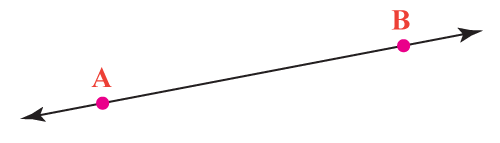A line AB is represented by

 $\overline{\text{AB}}$

While lines have no definite beginning or end, they are represented in our day-to-day lives with examples such as railway tracks or the freeway.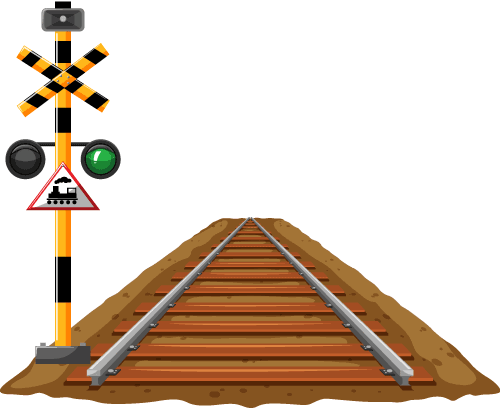### Ray

A ray is a part of a line that has only one fixed point and the other point does not have any end.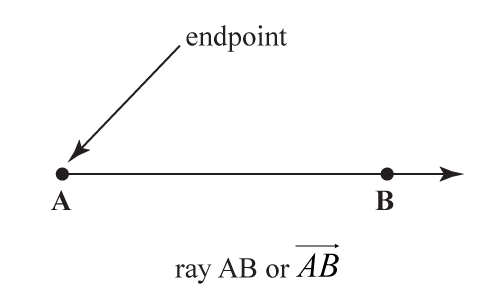A ray AB is represented by

 $\overrightarrow{\rm AB}$

While rays have a fixed beginning and no definite end, they are represented in our day-to-day lives with examples such as the sunlight or the light of a torch.### Segment

A segment is a part of a line that has a fixed length or we can say that both the ends of a segment are fixed. Segments, sometimes also referred to as line segments.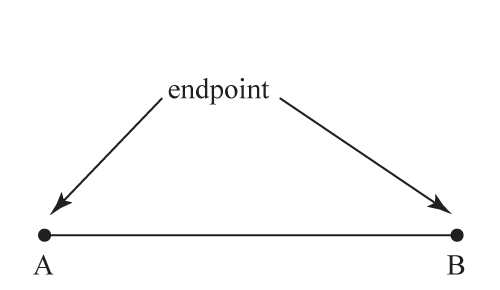A segment AB is represented by

 $\overline{\text{AB}}$

While line segments have both fixed ends, they are represented in our day-to-day lives with examples such as the edge of a table or some wire or pole.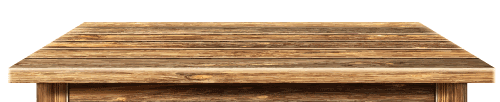Think Tank
• Let's suppose you tied a rope on one point on the Earth and started walking with the other end of the rope in your hand. You are walking in a straight line, after so many months you somehow managed to return to the same point from where you started. One of your friends points out that while you travelled in a line, the figure obtained by the rope was not a line. What answer do you give your friend?

## What Are Parallel Lines?

Two lines are said to be parallel lines if they lie in the same plane and never meet.

Or we can say that if two lines do not have any intersection point they are said to be parallel lines.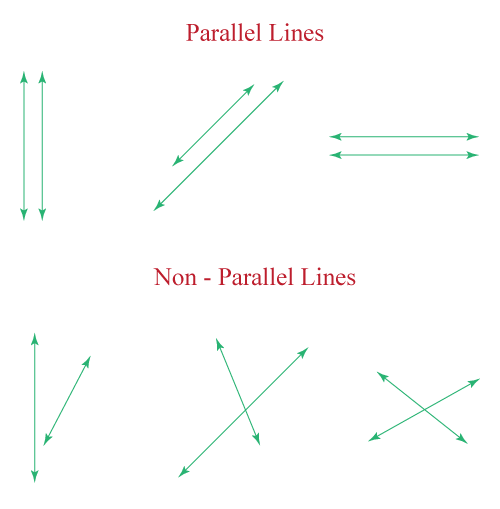### Intersecting Lines

If two lines meet at a point then they are said to be interesting lines.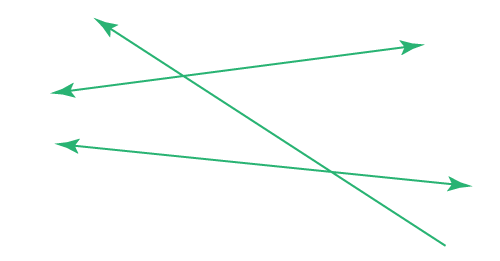## What Are Perpendicular Lines?

If two intersecting lines meet each other at $$90^{\circ}$$ angle, then they are said to be perpendicular lines.

Or if the angle between two lines is $$90^{\circ}$$ then they are perpendicular to each other.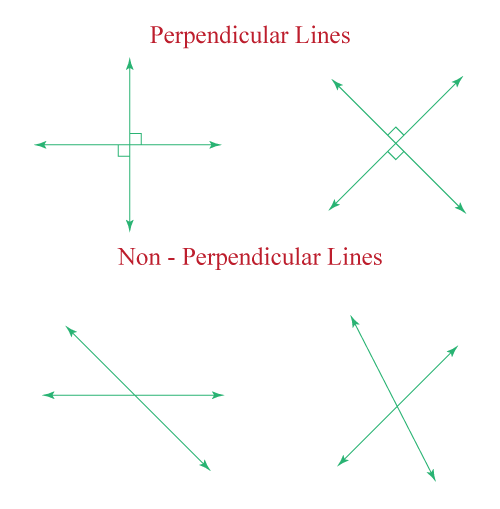Explore the types of lines in the below simulation.

## Horizontal And Vertical Lines

Lines are classified on the basis of their alignment.

### Horizontal Lines

The lines which are drawn horizontally are called horizontal lines.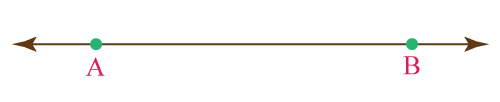### Vertical Lines

The lines which are drawn vertically are called vertical lines.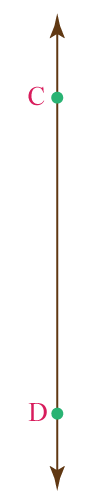Oblique or Slanting Lines

The lines which are drawn in a slanting position or it is forming some angle other than 0, 90, 180, 270, 360 degrees with the horizontal or vertical lines are called oblique or slanting line.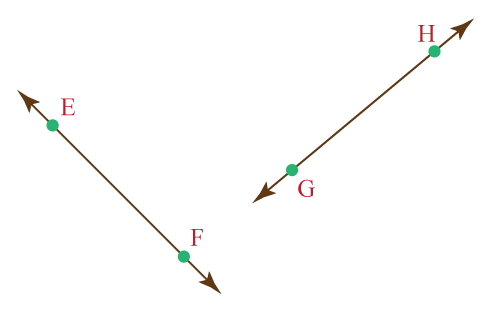## Solved Examples

 Example 1

Sam wants to find out the figures which are made up of line segments only. Help him to pick out the correct figures from the following.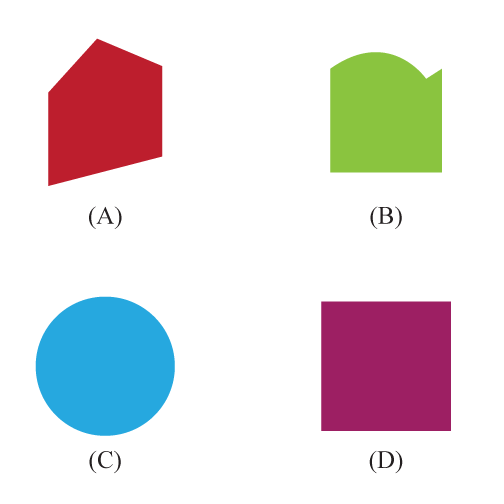Solution

Let's discuss each option one by one:

Option A is made up of 5 line segments.

Option B is made up of 3 line segments and a curved part.

Option C is a circle hance it is made up of only curved parts.

Option D is made up of 4 line segments.

 $$\therefore$$ Options A and D are correct.
 Example 2

Find the correct types of lines from the figure given below.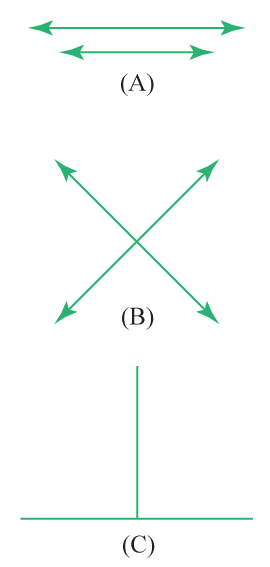Solution

Option A is a pair of parallel lines

Option B is a pair of non-parallel lines or intersection lines.

Option C is an example of perpendicular lines.

 Example 3

Tom is picking the points of intersection of the lines given in the figure below, he observed that there are 5 points of intersection. Is he right?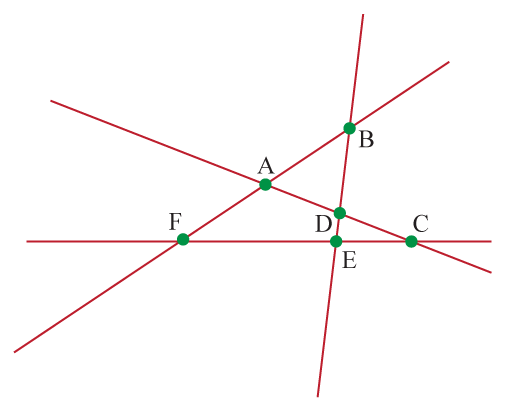Solution

The points at which any of these lines are intersecting are: $$\text{A, B, C, D, E, F}$$

Hence there are total 6 points of intersections.

 $$\therefore$$ Tom is not right

## Interactive Questions

Here are a few activities for you to practice.

## Let's Summarize

We hope you enjoyed learning about lines with the interactive questions. Now, you will be able to easily solve problems on lines.

At Cuemath, our team of math experts is dedicated to making learning fun for our favorite readers, the students!

Through an interactive and engaging learning-teaching-learning approach, the teachers explore all angles of a topic.

Be it worksheets, online classes, doubt sessions, or any other form of relation, it’s the logical thinking and smart learning approach that we, at Cuemath, believe in.

## FAQs on Lines

### 1. What is the equation of a line?

The general equation of a straight line can be given as $$ax + by + c= 0$$.

Where $$a, b, c$$ are constants and $$x, y$$ are variables.

### 2. What is the angle between two perpendicular lines?

The angle between the two perpendicular lines is 90 degrees.

### 3. What is a secant line?

A secant line is a line that intersects at two points with a circle.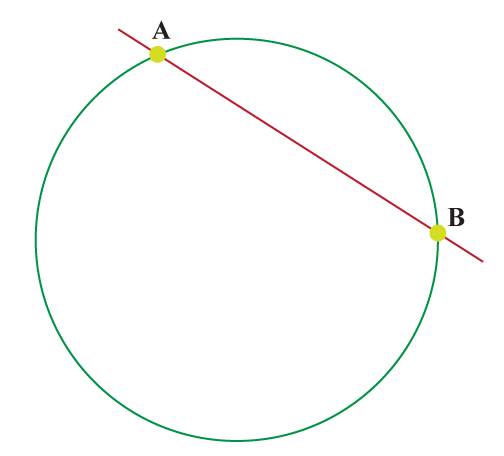The line AB is the secant to the circle.

### 4. What is a tangent line?

A tangent line is a line that touches the circle at one point.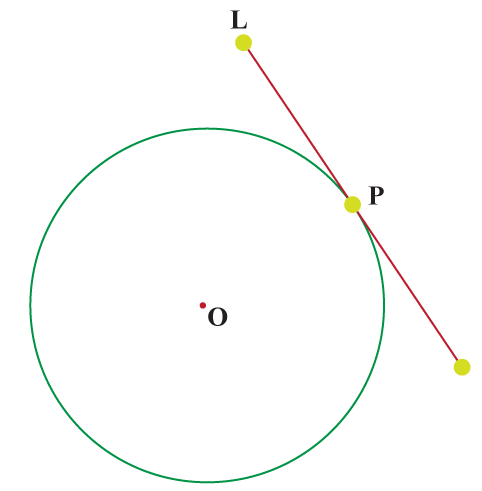The line $$L$$ is the tangent to the circle at point $$P$$.

### 5. What are the parallel lines?

Two lines are said to be parallel lines if they lie in the same plane and never meet.

Lines and Angles
Lines and Angles
Construction of Lines and Angles
Lines and Angles
Lines and Shapes
Lines, Line Segments, and Rays
Lines, Line Segments, and Rays
Geometry
Geometry
grade 9 | Questions Set 2
Geometry
Lines and Angles
Lines and Angles
Lines and Angles
Lines and Angles
Construction of Lines and Angles
Lines and Angles
Lines and Shapes
Geometry
grade 9 | Questions Set 1
More Important Topics
Numbers
Algebra
Geometry
Measurement
Money
Data
Trigonometry
Calculus
More Important Topics
Numbers
Algebra
Geometry
Measurement
Money
Data
Trigonometry
Calculus